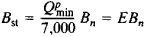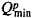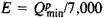# Fuel, Standard

## Fuel, Standard

an arbitrary unit used in calculations of organic fuels to compare efficiencies of different types of fuel and to make general evaluations. One kilogram of fuel with a heat of combustion of 7,000 Calories (kcal) per kg (29.3 megajoules per kg) is the unit of standard fuel used in the USSR. The ratio between the standard fuel and a natural fuel is given by the formulawhere Bst is the weight of an equivalent amount of standard fuel (in kg), Bn is the weight of the natural fuel (in kg for liquid and solid fuels or in m3 for gaseous fuels),is the minimum heat of combustion of the given natural fuel (in kcal/kg or kcal/m3), andis the caloric equivalent. The values of E are 1.4 for petroleum, 0.93 for coke, 0.4 for peat, and 1.2 for natural gas.

The concept of a standard fuel is especially convenient for comparing the costs of different thermal power installations. For example, the amount of standard fuel consumed to produce a unit of electric power is often used in calculations in power engineering. This value, g, is expressed in grams of standard fuel required to produce 1 kilowatt-hour of electric energy and is related to the efficiency η of the power installation by the formula g = 860/7η. The concept of a standard fuel is also useful in computing the fuel balance or total energy balance of an industry, a country, or the world.

In some countries, different values for a standard fuel have been adopted. In France, for example, the standard fuel is taken to have either a minimum heat of combustion of 6,500 kcal/kg (27.3 megajoules per kg) or a maximum heat of combustion of 6,750 kcal/kg (28.3 megajoules per kg). In the USA and Great Britain, a unit equal to 1018 British thermal units (36 billion tons of standard fuel) is taken as the large unit of standard fuel.

I. N. ROZENGAUZ

Site: Follow: Share:
Open / Close